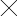﻿﻿﻿出国留学考试培训,留学语言考试培训,快速提分提高竞争力_托普仕留学Document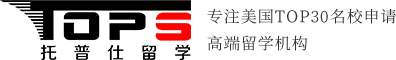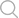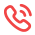#### 热线电话

(8:00~24:00)

400 - 686 - 9991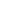当前位置：托普仕留学 >ACT >ACT数学

# act数学考试考察范围及注意事项

对于备战act考试数学部分的同学来讲，只有掌握act数学的考察范围，才可以在备考过程中更有针对性的进行复习!因此，本文，托普仕留学小编将就act数学考试考察范围：算术、初级代数、中级代数、几何坐标、平面几何、三角学，逐一进行介绍，还请大家能够重视!

act考试数学部分的时间为60分钟，考生需完成60道选择题。act数学考试从以下六个方面对考生进行考核：

1. 算术(Pre-Algebra)

考核的内容为高中之前学习的知识。例如，分数(fraction)、小数(decimal)、整数(integer)、平方根(square root)、比率(ratio)、百分比(percent)、整数的倍数(multiple)和因数(factor)、绝对值(absolute value)、一次方程式(linear equations with one variable)、概率(probability)等。

2. 初级代数(Elementary Algebra)

考察变量表达式(use variables to express relationships)、

代数表达式的代入法(substitute the value of a variable inan expression)、

解含有一个变量的线性不等式(solve linear inequalities with onevariable)、

应用指数和平方根(apply properties of integer exponentsand square roots)等。

算术(Pre-Algebra)和初级代数(ElementaryAlgebra)两部分共24道题目。

3. 中级代数(Intermediate Algebra)

4. 几何坐标(Coordinate Geometry)

考察标准x,y坐标平面(standard (x ,y) coordinate plane)中的点(point)、线(line)、多项式(polynomial)、圆(circle)、曲线(curve)、斜率(slope)、平行(parallel)、垂直(perpendicular)、距离(distance)、中点(midpoint)、变换(transformation)、二次曲线(conic)等。

中级代数(Intermediate Algebra)和几何坐标(Coordinate Geometry)共18道题目。

5. 平面几何(Plane Geometry)

考生需掌握平面图形的性质和关系，包括角(triangle)、矩形(rectangle)、平行四边形(parallelogram)、梯形(trapezoid)、圆(circle)、平行线(parallel line)、正交线(perpendicular line)、平移(translation)、旋转(rotation)、周长(perimeter)、面积(area)、体积(volume)等。

6. 三角学(Trigonometry)

考察直角三角形(righttriangle)、三角函数(trigonometric function)的值(value)、属性(property)和图形(graph)、三角恒等式(trigonometric identity)等。

平面几何(Plane Geometry)和三角学(Trigonometry)共18道题目。

以上就是托普仕留学小编为大家分享的act数学考试考察范围及注意事项的全部内容，希望对您有所帮助

*姓 名：
*性 别：
* 电 话：
* 邮 箱：
* 预咨询项目：
* 留言内容：
Document